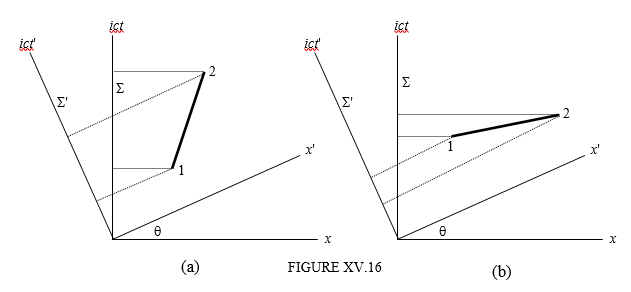$$\require{cancel}$$

# 15.14: Order of Events, Causality and the Transmission of Information

•• Contributed by Jeremy Tatum
• Emeritus Professor (Physics & Astronomy) at University of Victoria

Maybe it is even possible that if one event precedes another in one reference frame, in another reference frame the other precedes the one. In other words, the order of occurrence of events may be different in two frames. This indeed can be the case, and Minkowski diagrams (Figure XV.16) can help us to see why and in what circumstances.In part (a), of the two events 1 and 2, 1 occurs before 2 in either $$\sum$$ or $$\sum'$$. (from this point on I shall use a short phrase such as “in $$\sum$$” rather than the more cumbersome “when referred to the reference frame $$\sum$$”. But in part (b), event 1 occurs before event 2 in $$\sum$$, but after event 2 in $$\sum'$$. One can see that there is reversal of order of events if the slope of the line joining to two events is less than the angle $$\theta$$. The angle $$\theta$$, it may be recalled, is an imaginary angle such than $$\tan\theta=i\beta=\frac{i\nu}{c}$$, where $$\nu$$ is the relative speed of the two frames. In Figure XV.17, for simplicity I am going to suppose that event 1 occurs at the origin of both frames, and that event 2 occurs at coordinates ($$\nu\ t\ ,\ ict$$) in S. The condition for no reversal of events is then evidently

$\frac{ict}{\nu t}\geq \tan\theta=i\beta=\frac{i\nu}{c};$

or

$\nu \leq c \label{15.14.1}$

Now suppose that events 1 and 2 are causally connected in the sense that event 1 is the cause of event 2. For this to be the case, some signal carrying information must travel from 1 to 2. However, if event 1 is the cause of event 2, event 1 must precede event 2 in all reference frames. Thus it follows that no signal carrying information that could cause an event to occur can travel faster than the speed of light.

This means, in effect, that neither mass nor energy can be transmitted faster than the speed of light. That is not quite the same thing as saying that “nothing” can be transmitted faster than the speed of light. For example a Moiré pattern formed by two combs with slightly different tooth spacings can move faster than light if one of the combs is moved relative to the other; but then I suppose it has to be admitted that in that case “nothing” is actually being transmitted – and certainly nothing that can transmit information or that can cause an event. An almost identical example would be the modulation envelope of the sum of two waves of slightly different frequencies. A well-known example from wave mechanics is that of the wave representation of a moving particle. The wave group (which is the integral of a continuous distribution of wavelengths whose extent is governed by Heisenberg’s principle) moves with the particle at a sub-luminal speed, but there is nothing to prevent the wavelets within the group moving through the group at any speed. These wavelets may start at the beginning of the group and rapidly move through the group and extinguish themselves at the end. No “information” is transmitted from $$A$$ to $$B$$ at a speed any faster than the particle itself is moving.